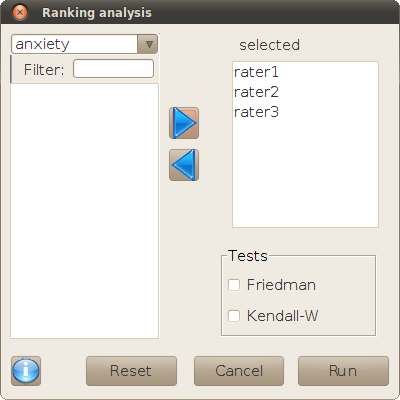## Description

This dialog is used for executing Friedman's or Kendall's W tests. These tests are nonparametric alternatives to repeated-measures ANOVA, specifically for unreplicated complete block designs. In such designs there are n random 'blocks' and p experimental conditions, so that all blocks are measured once in every condition. Thus, the data of the experiment may be arranged in a n×p table. Those data are ranked row-wise before computing and testing the statistics, so there is no specific assumption about how the data are distributed (that is why they are nonparametric tests).

The null hypotheses are presented by different statements for each test. In Friedman's original paper the hypothesis is that the expected ranks of all experimental conditions are equal to each other. In Kendall's paper the problem is expressed in terms of the agreement/disagreement between different persons when rating a series of items, and the null hypothesis is that there is no community of judgment. Nevertheless, both hypotheses are equivalent, although the calculations of each test are different. The main practical difference is that Kendall's test includes a procedure for correcting ties within raters. See also Friedman's paper comparing his and Kendall's methods in more detail.

## Dialog

The data must be arranged in a data frame with the 'blocks' or 'raters' in rows, and the experimental conditions or rated items in columns. If there are missing values in the data, the rows that contain them will be ignored. If the data are structured in the adequate matrix form, the only thing that must be done in the dialog is selecting the relevant columns and checking the boxes of the tests to be executed.## Operations with R code

If all the measures are in a single column, and the blocks and conditions coded as factors in other variables of the data frame, it will be necessary to transform the data frame beforehand. Let us suppose that the original data frame is called `dat`, with columns `measure`, `block`, and `condition` containing the information described by their names. The required transformation may be done by the function `cast` of package reshape as follows:

`dat2 <- cast(dat, block~condition, value="measure")`

Notice that the R functions that are called by these dialogs, `friedman.test` and `kendall` (the latter from package irr), take a different matrix. `friedman.test` uses a matrix with rows and columns as described in this document, whereas `kendall` uses a transposed version of that matrix (blocks in columns, and conditions in rows). This is done for the sake of coherence with other analyses covered in irr, where that structure is more adequate (e.g. `icc`). For the same reason, and further potential confusion, the documentation of `kendall` uses a nomenclature based on the 'rater's agreement' problem, where the the items evaluated by the raters are called by the rather ambiguous term 'subject'.